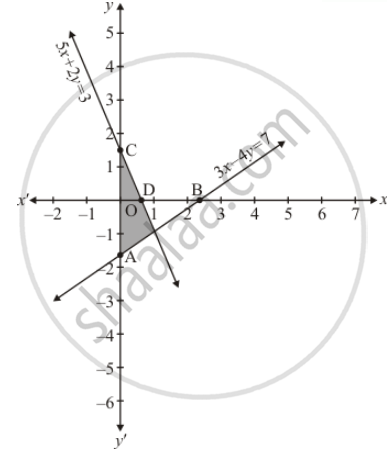# Solve the Following System of Equations Graphically: Shade the Region Between the Lines and the Y-axis 3x − 4y = 7 5x + 2y = 3 - Mathematics

Sum

Solve the following system of equations graphically:
Shade the region between the lines and the y-axis

3x − 4y = 7
5x + 2y = 3

#### Solution

The given equations are:

3x − 4y = 7  ......(i)

5x + 2y = 3  ........(ii)

Putting x = 0 in equation (i) weget

=> 3 xx 0 - 4y = 7

=> y = -7/4

x = 0, y = -7/4

Putting y  = 0 in eqaution (i) we get

=> 3x - 4 xx 0 = 7

=> x = 7/3

x= 7/3, y = 0

Use the following table to draw the graph.

 x 0 7/3 y -7/4 0

Draw the graph by plotting the two points A(0, -7/4), B(7/3, 0) from table.5x + 2y = 3 ....(ii)

Putting x = 0 in equation (ii) we get

=> 5 xx 0 + 2y = 3

=> y = 2/3

x = 3/5, y = 0

Use the following table to draw the graph

 x 0 3/5 y 3/2 0

Draw the graph by plotting the two points C(0, 3/2) and D(3/5, 0) from table.

The two lines intersect at points of y−axis.

Hence, x = 1and y = -1 is the Solution.

Concept: Graphical Method of Solution of a Pair of Linear Equations
Is there an error in this question or solution?

#### APPEARS IN

RD Sharma Class 10 Maths
Chapter 3 Pair of Linear Equations in Two Variables
Exercise 3.2 | Q 35.1 | Page 31